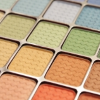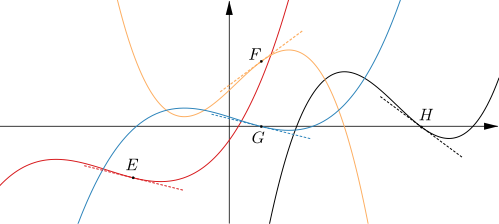#### You may also likeThis comes in two parts, with the first being less fiendish than the second. Itâ€™s great for practising both quadratics and laws of indices, and you can get a lot from making sure that you find all the solutions. For a real challenge (requiring a bit more knowledge), you could consider finding the complex solutions.### Discriminating

You're invited to decide whether statements about the number of solutions of a quadratic equation are always, sometimes or never true.This will encourage you to think about whether all quadratics can be factorised and to develop a better understanding of the effect that changing the coefficients has on the factorised form.

# Slippery Slopes

##### Age 16 to 18Challenge LevelThis resource is from Underground Mathematics.

Here are the graphs of four functions. The equations of the graphs are

$$y=f(x) \quad y=f(x)-8 \quad y=3f(x) \quad \text{and} \quad y=3f(x)+8.$$• The $x$-coordinates of $A$, $B$, $C$ and $D$ are all the same.  What can you deduce about the gradients of the curves at $A$, $B$, $C$ and $D$?
• The gradient of the tangent at $D$ is $\tfrac{1}{4}$. What are the gradients of the tangents at $A$, $B$ and $C$?

Here are the graphs of another four functions. The equations of these graphs are $$y=f(x)\quad y=f(x+20)-8 \quad y=3f(x-25) \quad \text{and} \quad y=-3f(x)+10.$$• The $x$-coordinates of points $E$, $F$, $G$ and $H$ are $-15$, $5$, $5$ and $30$ respectively. What can you deduce about these points?
• The gradient of the tangent at $E$ is $-\tfrac{1}{4}.$ What are the gradients of the tangents at $F$, $G$ and $H$?

This is an Underground Mathematics resource.

Underground Mathematics is hosted by Cambridge Mathematics. The project was originally funded by a grant from the UK Department for Education to provide free web-based resources that support the teaching and learning of post-16 mathematics.

Visit the site at undergroundmathematics.org to find more resources, which also offer suggestions, solutions and teacher notes to help with their use in the classroom.